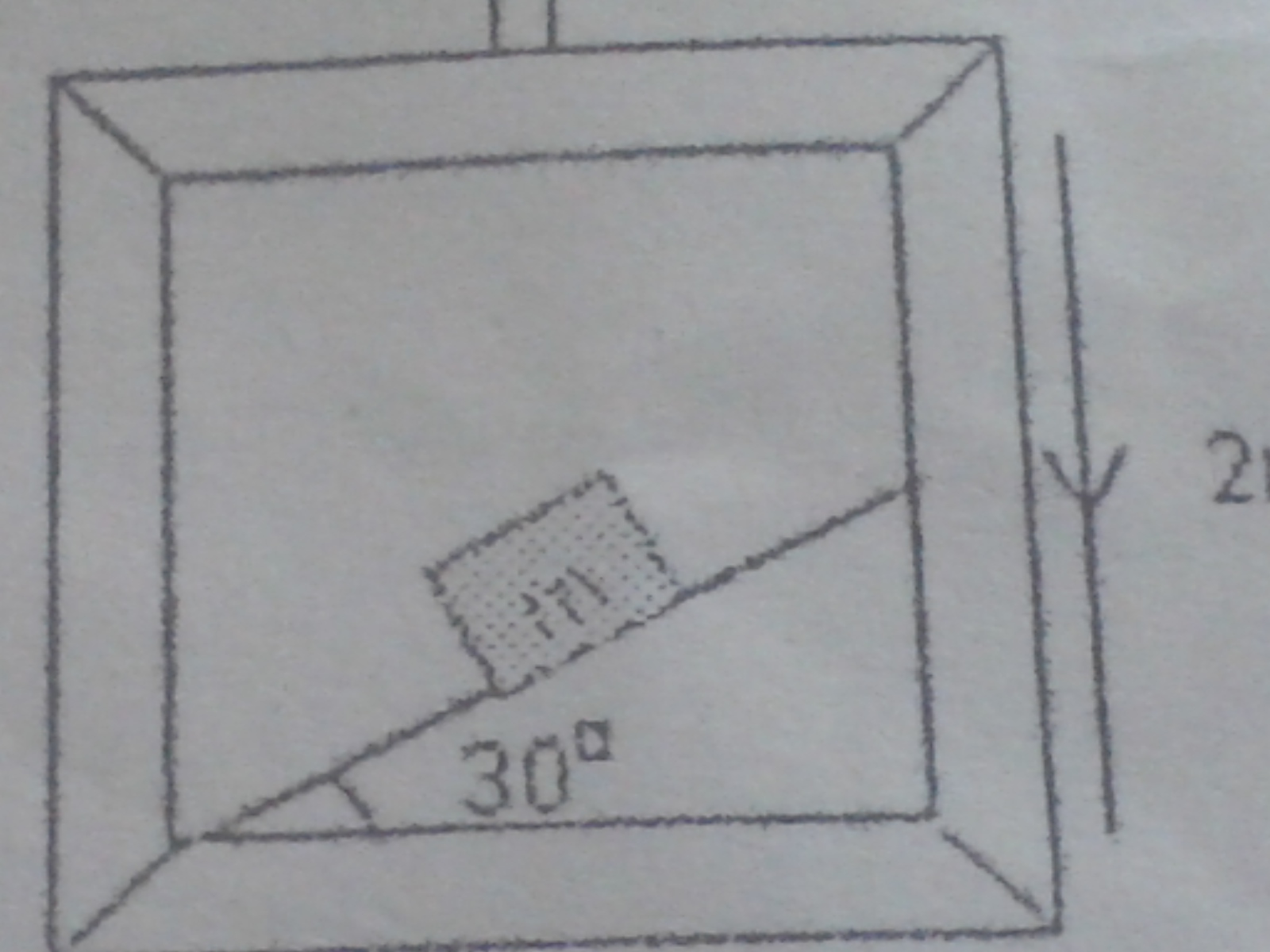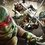# static frictionThis is a problem which I am confused and able to solve So please tell me how to solve

A block of mass market is kept on an incline plane of a lift moving down with acceleration of 2m/s^-2 . What should be the coefficient of friction for the block to move down with constant velocity relative to liftNote by Yogesh Ghadge
6 years, 7 months ago

This discussion board is a place to discuss our Daily Challenges and the math and science related to those challenges. Explanations are more than just a solution — they should explain the steps and thinking strategies that you used to obtain the solution. Comments should further the discussion of math and science.

When posting on Brilliant:

• Use the emojis to react to an explanation, whether you're congratulating a job well done , or just really confused .
• Ask specific questions about the challenge or the steps in somebody's explanation. Well-posed questions can add a lot to the discussion, but posting "I don't understand!" doesn't help anyone.
• Try to contribute something new to the discussion, whether it is an extension, generalization or other idea related to the challenge.
• Stay on topic — we're all here to learn more about math and science, not to hear about your favorite get-rich-quick scheme or current world events.

MarkdownAppears as
*italics* or _italics_ italics
**bold** or __bold__ bold
- bulleted- list
• bulleted
• list
1. numbered2. list
1. numbered
2. list
Note: you must add a full line of space before and after lists for them to show up correctly
paragraph 1paragraph 2

paragraph 1

paragraph 2

[example link](https://brilliant.org)example link
> This is a quote
This is a quote
    # I indented these lines
# 4 spaces, and now they show
# up as a code block.

print "hello world"
# I indented these lines
# 4 spaces, and now they show
# up as a code block.

print "hello world"
MathAppears as
Remember to wrap math in $$ ... $$ or $ ... $ to ensure proper formatting.
2 \times 3 $2 \times 3$
2^{34} $2^{34}$
a_{i-1} $a_{i-1}$
\frac{2}{3} $\frac{2}{3}$
\sqrt{2} $\sqrt{2}$
\sum_{i=1}^3 $\sum_{i=1}^3$
\sin \theta $\sin \theta$
\boxed{123} $\boxed{123}$

## Comments

Sort by:

Top Newest

We have to use concept of pseudo force here.

First we shall consider a reference frame which is moving along with lift. As it is non-inertial frame of reference so we have to include a pseudo force here.fig

Resolve the vectors in direction parallel to the plane and perpendicular to the plane. Then use newtons's second law to find equations.

If the block has to move with constant velocity then $F_{net}=0$.

Can you solve it from here?

- 6 years, 7 months ago

Log in to reply

Thanks you r the best

- 6 years, 7 months ago

Log in to reply

Thanks!

- 6 years, 7 months ago

Log in to reply

I solve as you told and I got the answer as 0.4 static friction can you tell this ans is right or wrong

- 6 years, 7 months ago

Log in to reply

Could you please show your steps. I am getting its value slightly differently.

- 6 years, 7 months ago

Log in to reply

Incline is at 30 ° so a= g sin 30°=5 So normal force = mg = 5m So the pseudo is acting as friction force here Then friction force (f)=static friction × normal force F=sf×5m 2m=sf×5m 2m/5m=sf 0.4= sf

- 6 years, 7 months ago

Log in to replyfig From the diagram

$N+2mcos\theta=mgcos\theta$............(1)

and $mgsin\theta=\mu N+2msin\theta$ ...................(2)

Find N from eq(1) and put its value in eq(2). Then find $\mu$

- 6 years, 7 months ago

Log in to reply

thank you for clearing the doubt

- 6 years, 7 months ago

Log in to reply

You are welcome. :D

- 6 years, 7 months ago

Log in to reply

×

Problem Loading...

Note Loading...

Set Loading...